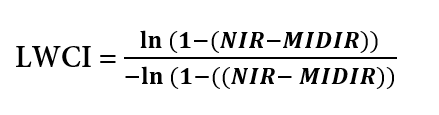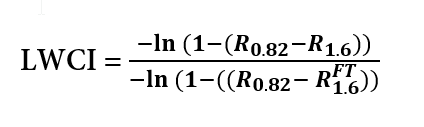# LWCI (leaf water content index)

Hi.

but nothing happend (I see all in black or white).

FB

Hello FB,

There is a comment in the script which says:

// colorBlend will return a color when the index is between min and max and white when it is less than min.
// To see black when it is more than max, uncomment the last line of colorBlend.
// The min/max values were computed automatically and may be poorly specified, feel free to change them to tweak the displayed range.

For example setting `min` and `max` to `-1.3` and `0.0` yields some results.

Hope it helps!

Kind regards,

Anze

Hi FB,

Based on the original articles about calculating LWCI, it seems that the equation is incorrect.
The equation from IndexDB is as follows:In the equation, R is the reflectance factor, where R_0.82 is reflectance at 0.82 µm → 820 nm (near-infrared) and R_1.6 is reflectance at 1.6 µm → 1600nm (mid-infrared).

As MIDIR means mid-infrared, it cannot be a mere number, as is in the current custom script, but should be a band. For sentinel-2, a mid-infrared band, corresponding to 1600 nm would be band 11.
Additionally, both logarithms should be negative, according to the article.

Also, if we look at the equation from the article, you see that (as opposed to the index DB equation) the second parameter in parenthesis of the denominator is different from the second parameter in parenthesis of the numerator; R_1.6_FT is reflectance at 1600nm at full turgor. Although I could not figure out how the full turgor was estimated, it could have something to do with research on the field:

" leaving only one parameter R_1.6_FT determined from a measured R_1.6 at a known RWC. "
(Article, page 45)

One idea I had was to suppose the R_1.6_FT is the maximum value of band 11, but the results were not satisfactory.
The following statement leads me to believe, that the LWCI could be multitemporal so that R_1.6_FT would mean the maximum reflectance values of band 11:

“The reflectanees of TM4 and TM5 at full turgor were used for TM4_FT and TM5_FT, then TM4 and TM5 measured on the other days were used to calculate LWCI.”
(Article, page 431)

*The articles used Landsat Thematic Mapper bands 4 and 5 (TM4, TM5).

As I am by no means an expert in plant science, I am not sure how the equation is supposed to be used with Sentinel-2 and if multitemporal approach would be correct.

If it helps, the normalized difference infrared index has (according to this article, page 45) a good correlation with canopy water content and it works:

index = (NIR - MIR) / (NIR + MIR)
index = (B08 - B11) / (B08 + B11)

Articles on LWCI:

1 Like